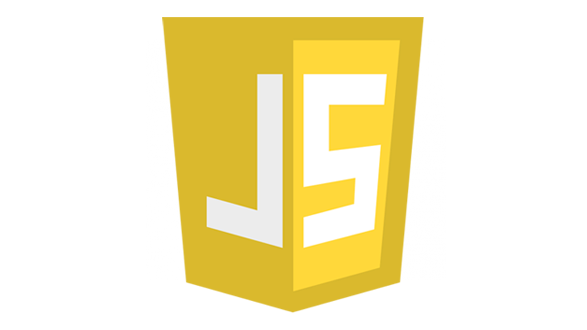# CSS - Measurement UnitsBefore we start the actual exercise, we would like to give a brief idea about the CSS Measurement Units. CSS supports a number of measurements including absolute units such as inches, centimeters, points, and so on, as well as relative measures such as percentages and em units. You need these values while specifying various measurements in your Style rules e.g. border = "1px solid red".

We have listed out all the CSS Measurement Units along with proper Examples −

Unit Description Example
% Defines a measurement as a percentage relative to another value, typically an enclosing element. p {font-size: 16pt; line-height: 125%;}
cm Defines a measurement in centimeters. div {margin-bottom: 2cm;}
em A relative measurement for the height of a font in em spaces. Because an em unit is equivalent to the size of a given font, if you assign a font to 12pt, each "em" unit would be 12pt; thus, 2em would be 24pt. p {letter-spacing: 7em;}
ex This value defines a measurement relative to a font's x-height. The x-height is determined by the height of the font's lowercase letter x. p {font-size: 24pt; line-height: 3ex;}
in Defines a measurement in inches. p {word-spacing: .15in;}
mm Defines a measurement in millimeters. p {word-spacing: 15mm;}
pc Defines a measurement in picas. A pica is equivalent to 12 points; thus, there are 6 picas per inch. p {font-size: 20pc;}
pt Defines a measurement in points. A point is defined as 1/72nd of an inch. body {font-size: 18pt;}
px Defines a measurement in screen pixels. p {padding: 25px;}

# CHOOSE ANY LANGUAGE